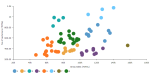# DCPY.MINIBATCHKMEANSCLUST(n_clusters, random_state, init, n_init, batch_size, max_no_improvement, max_iter, columns)

MiniBatch K-means is a variant of the K-means algorithm. It uses batches of data points to reduce the computation time, while attempting to optimize the same objective function (minimizing inertia or within-cluster sum-of-squares). Its main idea is to use small random batches of data points of a fixed size, where in each iteration a new random sample from the whole data set is obtained and used to recalculate the centroids. These steps are repeated until convergence.

###### Parameters
• n_clusters – Number of clusters that the algorithm should find, integer (default 8).
• random_state – Seed used to generate random numbers, integer (default 0).
• init – Method for initializing the centroids (default k-means++):
• k-means++ – First initial centroid is selected randomly, other ones are selected so they are as far apart from each other as possible. This method speeds up the convergence and helps to avoid converging at local optimum.
• random – Centroids are initially chosen randomly from input data points.
• n_init – Number of random initializations, integer (default 3).

• batch_size – Size of the mini batches, integer (default 100).

• max_no_improvement – Number of mini batches that do not improve clustering result, to control early stopping, integer (default 10).

• max_iter – Maximum number of iterations, integer (default 100).

• columns – Dataset columns or custom calculations.

Example: DCPY.MINIBATCHKMEANSCLUST(8, 0, 'k-means++', 3, 100, 10, 100, sum([Gross Sales]), sum([No of customers])) used as a calculation for the Color field of the Scatterplot visualization.###### Input data
• Numeric variables are automatically scaled to zero mean and unit variance.
• Character variables are transformed to numeric values using one-hot encoding.
• Dates are treated as character variables, so they are also one-hot encoded.
• Size of input data is not limited, but many categories in character or date variables increase rapidly the dimensionality.
• Rows that contain missing values in any of their columns are dropped.
###### Result
• Column of integer values starting with 0, where each number corresponds to a cluster assigned to each record (row) by the MiniBatch K-means algorithm.
• Rows that were dropped from input data due to containing missing values have missing value instead of an assigned cluster.
###### Key usage points
• When compared to K-means, this algorithm reduces the computation time. The time reduction is noticeable especially on bigger data sets and when a higher number of clusters is present.
• More efficient memory usage.
• When compared to K-means, this algorithm provides a lower quality of cluster separation (however, the loss in quality is not significant).
###### Example

For the whole list of algorithms, see Data science built-in algorithms.